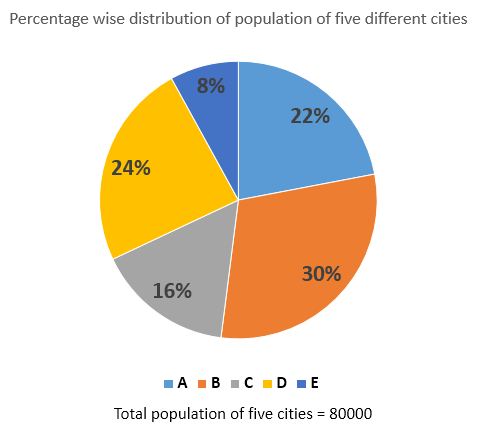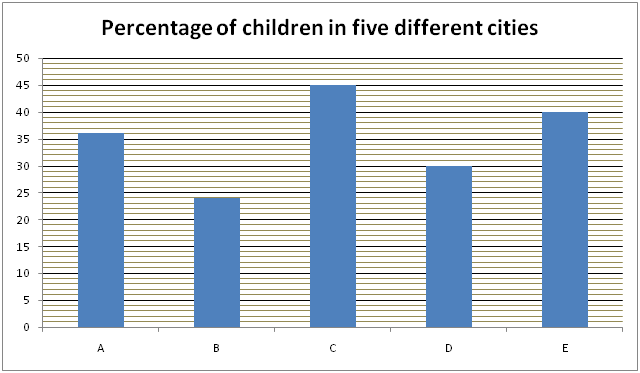# IBPS RRB PO Mains Quantitative Aptitude Questions 2019 (Day-11)

Dear Aspirants, Our IBPS Guide team is providing new series of Quantitative Aptitude Questions for IBPS RRB PO Mains 2019 so the aspirants can practice it on a daily basis. These questions are framed by our skilled experts after understanding your needs thoroughly. Aspirants can practice these new series questions daily to familiarize with the exact exam pattern and make your preparation effective.

Check here for IBPS RRB PO Mains Mock Test 2019

Check here for IBPS RRB Clerk Mains Mock Test 2019

Check here for IBPS PO Prelims Mock Test 2019

### Click Here to Subscribe Crack High Level Puzzles & Seating Arrangement Questions PDF 2019 Plan

[WpProQuiz 7129]

Directions (1 – 5): Study the following information carefully and answer the questions given below.1) Out of the adult population of city A, ratio of males and females is 6:5 respectively, number of adult males in city A is approximately what percent of the number of children in that city?

a) 79%

b) 83%

c) 87%

d) 91%

e) 97%

2) Find the respective ratio of number of children in city B and number of children in city C.

a) 1:2

b) 1:1

c) 2:3

d) 3:4

e) None of these

3) Find the difference between number of adults in city C and number of adults in city D.

a) 6400

b) 3600

c) 5200

d) 4800

e) None of these

4) Out of the adult population of city E, 20% of the population is female and rest are males. Number of adult males is what percent more/less than the number of children in that city?

a) 20% less

b) 20% more

c) 15% less

d) 25% more

e) None of these

5) Find the sum of total number of children in all the cities.

a) 30124

b) 28144

c) 26176

d) 32142

e) None of these

Directions (6 – 10): In each of these questions, one term in the given number series is wrong. Find out the wrong term.

6) 32, 31, 58, 160, 644, 3195

a) 58

b) 160

c) 644

d) 31

e) None of these

7) 2, 3, 9, 54, 403, 3612

a) 3

b) 403

c) 9

d) 54

e) None of these

8) 4, 4, 18, 65, 266.5, 1241.25

a) 18

b) 65

c) 266.5

d) 4

e) None of these

9) 6, 14, 42, 135, 517, 2377.5

a) 42

b) 517

c) 135

d) 14

e) None of these

10) 11, 24, 40, 56, 75, 96

a) 75

b) 40

c) 56

d) 24

e) None of these

Directions (1-5) :

Total population of city A = 22/100 x 80000 = 17600

Adult population of city A = (100 – 36)/100 x 17600

= 64/100 x 17600

= 11264

Number of adult males = 6/11 x 11264 = 6144

Number of children in city A = 36/100 x 17600 = 6336

Required percentage = 6144/6336 x 100 = 96.96% = 97% approx.

Total population of city B = 30/100 x 80000 = 24000

Total number of children in city B = 24/100 x 24000 = 5760

Total population of city C = 16/100 x 80000 = 12800

Total number of children in city C = 45/100 x 12800 = 5760

Required ratio = 5760:5760 = 1:1

Total population of city C = 16/100 x 80000 = 12800

Number of adults in city C = (100 – 45)/100 x 12800

= 55/100 x 12800

= 7040

Total population of city D = 24/100 x 80000 = 19200

Number adults in city D = (100 – 30)/100 x 19200

= 70/100 x 19200

= 13440

Required difference = 13440 – 7040 = 6400

Total population of city E = 8/100 x 80000 = 6400

Adult population of city E = (100 – 40)/100 x 6400

= 60/100 x 6400

= 3840

Number of adult males in city E = (100 – 20)/100 x 3840

= 80/100 x 3840

= 3072

Number of children in city E = 40/100 x 6400 = 2560

Required percentage = (3072 – 2560)/2560 x 100

= 512/2560 x 100

= 20% more

Total population of city A = 22/100 x 80000 = 17600

Number of children in city A = 36/100 x 17600 = 6336

Total population of city B = 30/100 x 80000 = 24000

Number of children in city B = 24/100 x 24000 = 5760

Total population of city C = 16/100 x 80000 = 12800

Number of children in city C = 45/100 x 12800 = 5760

Total population of city D = 24/100 x 80000 = 19200

Number of children in city D = 30/100 x 19200 = 5760

Total population of city E = 8/100 x 80000 = 6400

Number of children in city E = 40/100 x 6400 = 2560

Required sum = 6336 + 5760 + 5760 + 5760 + 2560 = 26176

Directions (6-10) :

(32 – 1) x 1 = 31

(31 – 2) x 2 = 58

(58 – 3) x 3 = 165 (not 160)

(165 – 4) x 4 = 644

(644 – 5) x 5 = 3195

2 x 5 – 7 = 3

3 x 6 – 9 = 9

9 x 7 – 11 = 52 (not 54)

52 x 8 – 13 = 403

403 x 9 – 15 = 3612

4 x 0.5 + 2 = 4

4 x 1.5 + 12 = 18

18 x 2.5 + 22 = 67 (not 65)

67 x 3.5 + 32 = 266.5

266.5 x 4.5 + 42 = 1241.25

6 x 0.5 + 11 = 14

14 x 1.5 + 21 = 42

42 x 2.5 + 31 = 136 (not 135)

136 x 3.5 + 41 = 517

517 x 4.5 + 51 = 2377.5

1 x 11 = 11

2 x 12 = 24

3 x 13 = 39 (not 40)

4 x 14 = 56

5 x 15 = 75

6 x 16 = 96